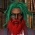Display replies flat, with oldest first Display replies flat, with newest first Display replies in threaded form Display replies in nested formJean-Dominique Deuschel (Technische Universität Berlin) "The Random Conductance Model"by Egor Pifagorov - Sunday, 7 December 2014, 09:08 PM Коллоквиум лаборатории Чебышева Четверг 18 декабря в 17:15 в ауд. 14 (14-я линия В.О., 29) Jean-Dominique Deuschel (Technische Universität Berlin) The Random Conductance ModelThe random conductance model describes the erratic motion {X_n, n>=0} of a particle on the d-dimensional lattice Z^d. The random walk X_n is a Markov process which jumps at discrete times n from x \in Z^d to a nearest neighbor y according to a probability 0< p(x,y) <= 1. In the classical setting these transition probabilities are homogeneous that is p(x,y)=p(x-y,0)=p(0,y-x). One is interested in the long time behavior of the walk. A famous theorem of Donsker shows that walk behaves diffusively, that is at time N^2 the walk travels to a distance N, so that the rescaled process {X^{(N)}_t=X_{[N^2t]}/N, t >= 0} converges to a homogeneous diffusion process: the Brownian motion, or Wiener process. In the Random Conductance Model the above homogeneous transition probabilities p(x,y)=p(x-y,0) are replaced by symmetric random probabilities omega(x,y)=omega(y,x). The corresponding random walk x_n is thus evolving in a highly disordered media. However if the distribution of the random media is assumed to be ergodic, one expects that along the trajectories of the random walk, homogenization takes place, in particular that the rescaled process X^{(N)} also converges to the Brownian motion. In fact this has been proved un the uniformly elliptic case, when the transition probabilities are bounded from below by Kozlov and Kipnis-Varadhan. In the general degenerate unbounded case, the random walk might get trapped at sites with very small the transition probabilities, so that moment conditions are required for the limit theorem. We will explain some of the probabilistic arguments, based on the ergodic theorem and martingalesand some of the analytical tools relying on harmonic analysis and Sobolev inequalities.